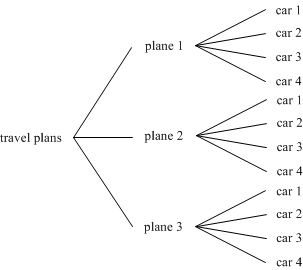x2
Introduction
( )
Types of Arrangements
=
Two More Methods
=
Some Applications

# Section 1.2 – Two Better Methods (Part 1)

There's a restaurant up the street from my office that has a machine called the Coca Cola Freestyle that lets people "build their own soda". You tell it the type of soda that you want and what kind of flavoring you want and it mixes the soda you selected into a cup. You can go traditional with a cherry Coke or have something avant garde like an orange Dr. Pepper. If the machine has 13 different types of soda and 9 different flavorings, how many different drinks will it make?

This question shows the problem with our tree diagrams. The quick answer is, a lot. Trying to make a tree diagram would take quite a bit of time and paper. Also, when you start to make trees that big, the possibility of making a mistake somewhere in the process starts to increase and that's one of the things our tree diagrams were supposed to help prevent. If we're going to handle problems with bigger numbers like the Freestyle machine, we're going to need some better tools.

## The Addition Rule

Suppose you need to go from Tampa to Louisville. You can do this by one of three different plane flights or by choosing one of two rental car options. How many ways can you make the trip? Think about this for a minute before you continue reading and see if you can come up with a number.

This brings us to our first counting rule.

The Addition Rule
If a single task can be done in one of m ways or one of n ways and the two sets of ways have no options in common then there are n + m ways to do the task.

To see how you use this rule, let's walk through all of its parts and see how it applies to our travel question.

1. We have exactly one task or choice to make: picking a way to make the trip.
2. There are two groups from which we can choose: planes and rental cars.
3. None of the options in the first category (planes) is also in the second category (cars).

Because all of those requirements have been met we can use the Addition Rule to find the number of travel options. Because there are 3 options in the first group (plane flights) and 2 options in the second group (rental cars), the Addition Rule tells us that the total number of travel options is 3 + 2 = 5.In this case, we can confirm the result by listing all of the possible options:

plane flight 1 or plane flight 2 or plane flight 3 or rental car 1 or rental car 2

You can see from the list that the total number of travel options is five, i.e. three plane options + two car options, which corresponds with what the Addition Rule tells us.

This rule may seem a little trivial at first. While it doesn't get used as much as the Multiplication Rule that we'll talk about next, it's a tool that you should have in your back pocket. In the cases where it can be used, it makes answering questions a lot simpler.

## The Multiplication RuleThinking about our traveler again, suppose that he decides to fly. He's still going to need a vehicle when he gets to Louisville so he'll have to rent a car. If he still has the 3 plane flights to choose from and 4 rental car choices when he lands, how many different travel combinations does he have?

We can't use the Addition Rule here because we have more than one choice to make. Our traveler has to choose both a plane and a rental car. To start thinking about this situation, I'm going to draw out the tree diagram. You can see what it looks like to the right. Notice how the first set of branches takes the one "travel plans" option and splits it into three, i.e. it triples the number of branches.

Now look at what the car options do. Each set of car branches takes a plane branch and splits it into four branches. This turned the original three plane options into 3 · 4 or 12 plane/car combinations.

This splitting process where every new choice multiplies the total number of branches brings us to our second counting rule.

The Multiplication Rule
If a task requires two steps, the first step can be done in m ways and the second step can be done in n ways then there are m · n ways that the task can be completed.

Let's think about how this applies to our traveler situation.

1. We're making one choice that requires two steps: choosing the plane and choosing a car.
2. The first choice, picking the plane, can be done in m = 3 ways.
3. The second choice, picking a car, can be done in n = 4 ways.

The Multiplication Rule tells us that we must have m · n = 3 · 4 = 12 different travel options which matches what we got from our tree diagram.

This rule tells us what to do in the case of a task with two steps, however, it works for tasks with more than two parts. No matter how many parts or choices there are, you can find the total number of ways a task can be completed by multiplying together the number of ways that its individual steps can be completed.

# Videos

In the second part of this section we'll look at some specific examples and some practice exercises.